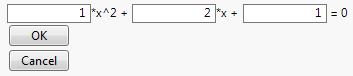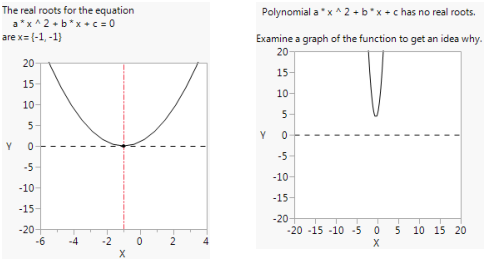Scripting Guide > Display Trees > Construct Custom Windows > Example of Constructing a Custom Platform
Publication date: 11/10/2021

## Example of Constructing a Custom Platform

An example in Manipulating Expressions, showed how to use the Substitute Into() function to input coefficients for a quadratic polynomial into the quadratic formula and then use the formula to calculate the roots of the polynomial. That example required specifying the coefficients as arguments to Substitute Into().

The section Construct a Column Dialog shows an example to collect coefficients from the user using a modal dialog box.

This section further develops the example into a complete customized platform that first displays a dialog box to ask for coefficients, finds the roots, and then displays the results along with a graph in a custom window.

// create a window to collect coefficients from the user

`myCoeffs = New Window( "Find the roots for the equation",`
`	<<Modal,`
`	H List Box(`
`		a = Number Edit Box( 1 ),`
`		Text Box( "*x^2 + " ),`
`		b = Number Edit Box( 2 ),`
`		Text Box( "*x + " ),`
`		c = Number Edit Box( 1 ),`
`		Text Box( " = 0" )`
`	),`
`	Button Box( "OK",`
`		a = a << Get;`
`		b = b << Get;`
`		c = c << Get;`
`		Show( a, b, c );`
`	),`
`	Button Box( "Cancel" )`
`);`
` `

/* calculate the results: The quadratic formula is

x=(-b + - sqrt(b^2 - 4ac))/2a. Plug the coefficients into

`x = {Expr(`
`	(-b + Sqrt( b ^ 2 - 4 * a * c )) / (2 * a)`
`), Expr(`
`	(-b - Sqrt( b ^ 2 - 4 * a * c )) / (2 * a)`
`)};`
`xx = Eval Expr( x );`
` `
` `
`results = Expr( // store the solution list`

/* test whether real roots were found and make an appropriate display

if yes (for example, with the window's defaults), show roots and a graph */

`	xmin = xx - 5;`
`	xmax = xx + 5;`
`	ymin = -20;`
`	ymax = 20;`
`	win = New Window( "The roots of a quadratic function",`
`		V List Box(`
`			Text Box( "The real roots for the equation " ),`
`			Text Box( "    " || Expr( po ) || " = 0" ),`
`			H List Box( Text Box( "are x=" ), Text Box( xxx ) ),`
`			Text Box( " " ), // to get a blank line`
`			Graph Box(`
`				Frame Size( 200, 200 ),`
`				X Scale( xmin, xmax ),`
`				Y Scale( ymin, ymax ),`
`				Line Style( 2 ),`
`				H Line( 0 ),`
`				Line Style( 0 ),`
`				Y Function( polynomial, x ),`
`				Line Style( 3 ),`
`				Pen Color( 3 ),`
`				V Line( xx ),`
`				V Line( xx ),`
`				Marker Size( 2 ),`
`				Marker( 0, {xx, 0}, {xx, 0} )`
`			)`
`		)`
`	);`
`);`
`error = Expr(`
` `

/* if no (for example, with a=3, b=4, c=5), put up an error

window with a helpful graph */

`	win = New Window( "Error",`
`		V List Box(`
`			Text Box( " " ),`
`			Text Box( "  Polynomial " || po || " has no real roots.  " ),`
`			Text Box( " " ),`
`			Text Box( "Examine a graph of the function to get an idea why." ),`
`			Graph Box(`
`				Frame Size( 200, 200 ),`
`				X Scale( -20, 20 ),`
`				Y Scale( -20, 20 ),`
`				Line Style( 2 ),`
`				H Line( 0 ),`
`				Line Style( 0 ),`
`				Y Function( polynomial, x )`
`			)`
`		)`
`	)`
`);`
` `

/* either way, the script needs to have some strings ready

rewrite the polynomial with the coefficients specified */

`polynomial = Expr( a * x ^ 2 + b * x + c );`
` `

// store this instance of the polynomial as a string

`po = Char( Eval Expr( polynomial ) );`
` `

// store the solution list as a string

`xxx = Char( Eval Expr( x ) );`
` `
`If( Is Missing( xx ) | Is Missing( xx ), // now it’s ready for a test`
`	error,`
`	results`
`);`

When you run the preceding script, you first see a window like this:

Figure 11.32 A Custom Platform Launch WindowClicking OK, displays a results window (Figure 11.33, left), with either the roots or an error message. Rerun the script, input 5, 4, and 5 respectively and click OK. Note that JMP displays an error message (Figure 11.33, right).

Figure 11.33 The Custom Platform’s Report